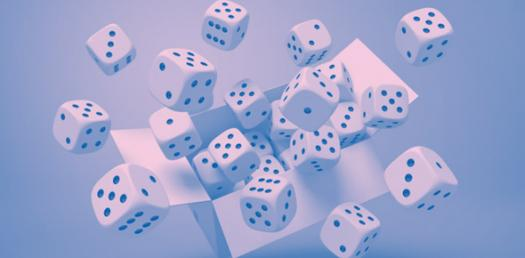# 10 - Maths - Unit 12. Probability

20 Questions | Attempts: 2945
ShareSettingsPrepared by Mr. A. GANESAN, VICE PRINCIPAL, SHRI GURU BRAMMA VIDHYALAYA, KARUR-5   ; &nbs p; & www. Padasalai. Net

• 1.
If S is the sample space of a random experiment, then P(S) =
• A.

1

• B.

0

• C.

1/8

• D.

1/2

• 2.
If p is probability of an event A, then p satisfies =
• A.

0 ≤ p ≤ 1

• B.

0 < p < 1

• C.

0 ≤ p < 1

• D.

0 < p ≤ 1

• 3.
Let A and B be any two events and S be the corresponding sample space. Then P(Ā ∩B)=
• A.

P(B)- P(A∩B)

• B.

P (A ∩B)- P(B)

• C.

P(S)

• D.

P[ (AUB)']

• 4.
The Probability that a student will score centum in mathematics is 4/5. The Probability that he will not score centum is =
• A.

1/5

• B.

2/5

• C.

3/5

• D.

4/5

• 5.
If A and B are two events such that P(A) = 0.25, P(B) = 0.05 and P(A∩B)=0.14, then P(AUB) =
• A.

0.16

• B.

0.61

• C.

0.14

• D.

0.6

• 6.
There are 6 defective items in a sample of 20 items. One item is drawn at random. The Probability that it is a non-defective item is
• A.

7/10

• B.

0

• C.

3/10

• D.

2/3

• 7.
If A and B are mutually exclusive events and S is the Sample space such that  P(A) =1/3P(B) and S= AUB, then P(A)=
• A.

1/4

• B.

1/2

• C.

3/4

• D.

3/8

• 8.
The Probabilities of three mutually exclusive events A, B  and C are given by 1/3,1/4 and 5/12. Then P(AUBUC)  is
• A.

1

• B.

19/12

• C.

11/12

• D.

7/12

• 9.
If P(A)= 0.25 , P(B)= 0.50 , P(AB) = 0.14 then  P(neither A or B ) =
• A.

0.39

• B.

0.25

• C.

0.11

• D.

0.24

• 10.
A bag contains 5 black balls , 4 white balls and 3 red balls. If a ball is selected at random , the probability that it is not red is =
• A.

3/4

• B.

5/12

• C.

4/12

• D.

3/12

• 11.
Two dice are thrown simultaneously. The probability of getting a doublet is =
• A.

1/6

• B.

1/36

• C.

1/3

• D.

2/3

• 12.
A fair die is thrown once . The probability of getting a prime or composite number is
• A.

5/6

• B.

1

• C.

0

• D.

1/6

• 13.
Probability of getting 3 heads or 3 tails in tossing a coin 3 times is
• A.

1/4

• B.

1/8

• C.

3/8

• D.

1/2

• 14.
A card is drawn from a pack of 52 cards at random. The probability of getting neither an ace  nor a king card is
• A.

11/13

• B.

2/13

• C.

4/13

• D.

8/13

• 15.
The probability that a leap year will have 53 fridays or 53 saturdays is
• A.

3/7

• B.

2/7

• C.

1/7

• D.

4/7

• 16.
The Probability that a non-leap year will have 53 Sundays and 53 Mondays is
• A.

0

• B.

1/7

• C.

2/7

• D.

3/7

• 17.
The Probability of selecting a queen of hearts when a cards is drawn from a pack of 52 playing cards is
• A.

1/52

• B.

16/52

• C.

1/13

• D.

1/26

• 18.
The Probability of sure event is
• A.

1

• B.

0

• C.

100

• D.

0.1

• 19.
The outcome of a random experiment results in either success or failure. If the probability  of success is twice the probability of failure, then the probability of success is
• A.

2/3

• B.

1/3

• C.

1

• D.

0

• 20.
If Ø is an impossible event, then  P(Ø) =
• A.

0

• B.

1

• C.

1/4

• D.

1/2

## Related TopicsBack to top
×

Wait!
Here's an interesting quiz for you.